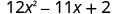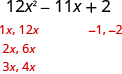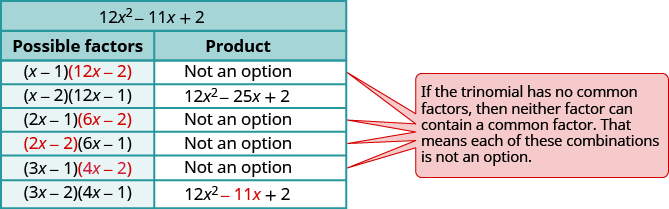# 7.5 General strategy for factoring polynomials

 Page 1 / 2
By the end of this section, you will be able to:
• Recognize and use the appropriate method to factor a polynomial completely

Before you get started, take this readiness quiz.

1. Factor ${y}^{2}-2y-24$ .
If you missed this problem, review [link] .
2. Factor $3{t}^{2}+17t+10$ .
If you missed this problem, review [link] .
3. Factor $36{p}^{2}-60p+25$ .
If you missed this problem, review [link] .
4. Factor $5{x}^{2}-80$ .
If you missed this problem, review [link] .

## Recognize and use the appropriate method to factor a polynomial completely

You have now become acquainted with all the methods of factoring that you will need in this course. (In your next algebra course, more methods will be added to your repertoire.) The figure below summarizes all the factoring methods we have covered. [link] outlines a strategy you should use when factoring polynomials.

## Factor polynomials.

1. Is there a greatest common factor?
• Factor it out.
2. Is the polynomial a binomial, trinomial, or are there more than three terms?
• If it is a binomial:
Is it a sum?
• Of squares? Sums of squares do not factor.
• Of cubes? Use the sum of cubes pattern.
Is it a difference?
• Of squares? Factor as the product of conjugates.
• Of cubes? Use the difference of cubes pattern.
• If it is a trinomial:
Is it of the form ${x}^{2}+bx+c$ ? Undo FOIL.
Is it of the form $a{x}^{2}+bx+c$ ?
• If $a$ and $c$ are squares, check if it fits the trinomial square pattern.
• Use the trial and error or “ac” method.
• If it has more than three terms:
Use the grouping method.
3. Check.
• Is it factored completely?
• Do the factors multiply back to the original polynomial?

Remember, a polynomial is completely factored if, other than monomials, its factors are prime!

Factor completely: $4{x}^{5}+12{x}^{4}$ .

## Solution

$\begin{array}{ccccccc}\text{Is there a GCF?}\hfill & & & \text{Yes,}\phantom{\rule{0.2em}{0ex}}4{x}^{4}.\hfill & & & \hfill 4{x}^{5}+12{x}^{4}\hfill \\ & & & \text{Factor out the GCF.}\hfill & & & \hfill 4{x}^{4}\left(x+3\right)\hfill \\ \text{In the parentheses, is it a binomial, a}\hfill & & & & & & \\ \text{trinomial, or are there more than three terms?}\hfill & & & \text{Binomial.}\hfill & & & \\ \phantom{\rule{1em}{0ex}}\text{Is it a sum?}\hfill & & & & & & \text{Yes.}\hfill \\ \phantom{\rule{1em}{0ex}}\text{Of squares? Of cubes?}\hfill & & & & & & \text{No.}\hfill \\ \text{Check.}\hfill & & & & & & \\ \\ \phantom{\rule{1em}{0ex}}\text{Is the expression factored completely?}\hfill & & & & & & \text{Yes.}\hfill \\ \phantom{\rule{1em}{0ex}}\text{Multiply.}\hfill & & & & & & \\ \\ \\ \phantom{\rule{2.5em}{0ex}}4{x}^{4}\left(x+3\right)\hfill & & & & & & \\ \phantom{\rule{2.5em}{0ex}}4{x}^{4}·x+4{x}^{4}·3\hfill & & & & & & \\ \phantom{\rule{2.5em}{0ex}}4{x}^{5}+12{x}^{4}\phantom{\rule{0.2em}{0ex}}✓\hfill & & & & & & \end{array}$

Factor completely: $3{a}^{4}+18{a}^{3}$ .

$3{a}^{3}\left(a+6\right)$

Factor completely: $45{b}^{6}+27{b}^{5}$ .

$9{b}^{5}\left(5b+3\right)$

Factor completely: $12{x}^{2}-11x+2$ .

## SolutionIs there a GCF? No. Is it a binomial, trinomial, or are there more than three terms? Trinomial. Are a and c perfect squares? No, a = 12, not a perfect square. Use trial and error or the “ac” method. We will use trial and error here.Check.

$\phantom{\rule{2.5em}{0ex}}\left(3x-2\right)\left(4x-1\right)$

$\phantom{\rule{2.5em}{0ex}}12{x}^{2}-3x-8x+2$

$\phantom{\rule{2.5em}{0ex}}12{x}^{2}-11x+2\phantom{\rule{0.2em}{0ex}}✓$

Factor completely: $10{a}^{2}-17a+6$ .

$\left(5a-6\right)\left(2a-1\right)$

Factor completely: $8{x}^{2}-18x+9$ .

$\left(2x-3\right)\left(4x-3\right)$

Factor completely: ${g}^{3}+25g$ .

## Solution

$\begin{array}{ccccccc}\text{Is there a GCF?}\hfill & & & \text{Yes,}\phantom{\rule{0.2em}{0ex}}g.\hfill & & & \hfill {g}^{3}+25g\hfill \\ \text{Factor out the GCF.}\hfill & & & & & & \hfill g\left({g}^{2}+25\right)\hfill \\ \text{In the parentheses, is it a binomial, trinomial,}\hfill & & & & & & \\ \text{or are there more than three terms?}\hfill & & & \text{Binomial.}\hfill & & & \\ \phantom{\rule{1em}{0ex}}\text{Is it a sum ? Of squares?}\hfill & & & \text{Yes.}\hfill & & & \text{Sums of squares are prime.}\hfill \\ \text{Check.}\hfill & & & & & & \\ \\ \phantom{\rule{1em}{0ex}}\text{Is the expression factored completely?}\hfill & & & \text{Yes.}\hfill & & & \\ \phantom{\rule{1em}{0ex}}\text{Multiply.}\hfill & & & & & & \\ \phantom{\rule{2.5em}{0ex}}g\left({g}^{2}+25\right)\hfill & & & & & & \\ \phantom{\rule{2.5em}{0ex}}{g}^{3}+25g\phantom{\rule{0.2em}{0ex}}✓\hfill & & & & & & \end{array}$

Factor completely: ${x}^{3}+36x$ .

$x\left({x}^{2}+36\right)$

Factor completely: $27{y}^{2}+48$ .

$3\left(9{y}^{2}+16\right)$

Factor completely: $12{y}^{2}-75$ .

## Solution

$\begin{array}{ccccccc}\text{Is there a GCF?}\hfill & & & \text{Yes, 3.}\hfill & & & \hfill 12{y}^{2}-75\hfill \\ \text{Factor out the GCF.}\hfill & & & & & & \hfill 3\left(4{y}^{2}-25\right)\hfill \\ \text{In the parentheses, is it a binomial, trinomial},\hfill & & & & & & \\ \text{or are there more than three terms?}\hfill & & & \text{Binomial.}\hfill & & \\ \text{Is it a sum?}\hfill & & & \text{No.}\hfill & & & \\ \text{Is it a difference? Of squares or cubes?}\hfill & & & \text{Yes, squares.}\hfill & & & \hfill 3\left({\left(2y\right)}^{2}-{\left(5\right)}^{2}\right)\hfill \\ \text{Write as a product of conjugates.}\hfill & & & & & & \hfill 3\left(2y-5\right)\left(2y+5\right)\hfill \\ \text{Check.}\hfill & & & & & & \\ \\ \phantom{\rule{1em}{0ex}}\text{Is the expression factored completely?}\hfill & & & \text{Yes.}\hfill & & & \\ \phantom{\rule{1em}{0ex}}\text{Neither binomial is a difference of}\hfill & & & & & & \\ \phantom{\rule{1em}{0ex}}\text{squares.}\hfill & & & & & & \\ \phantom{\rule{1em}{0ex}}\text{Multiply.}\hfill & & & & & & \\ \phantom{\rule{2.5em}{0ex}}3\left(2y-5\right)\left(2y+5\right)\hfill & & & & & & \\ \phantom{\rule{2.5em}{0ex}}3\left(4{y}^{2}-25\right)\hfill & & & & & & \\ \phantom{\rule{2.5em}{0ex}}12{y}^{2}-75\phantom{\rule{0.2em}{0ex}}✓\hfill & & & & & & \end{array}$

Aziza is solving this equation-2(1+x)=4x+10
No. 3^32 -1 has exactly two divisors greater than 75 and less than 85 what is their product?
x^2+7x-19=0 has Two solutions A and B give your answer to 3 decimal places
3. When Jenna spent 10 minutes on the elliptical trainer and then did circuit training for20 minutes, her fitness app says she burned 278 calories. When she spent 20 minutes onthe elliptical trainer and 30 minutes circuit training she burned 473 calories. How manycalories does she burn for each minute on the elliptical trainer? How many calories doesshe burn for each minute of circuit training?
.473
Angelita
?
Angelita
John left his house in Irvine at 8:35 am to drive to a meeting in Los Angeles, 45 miles away. He arrived at the meeting at 9:50. At 3:30 pm, he left the meeting and drove home. He arrived home at 5:18.
p-2/3=5/6 how do I solve it with explanation pls
P=3/2
Vanarith
1/2p2-2/3p=5p/6
James
Cindy
4.5
Ruth
is y=7/5 a solution of 5y+3=10y-4
yes
James
Cindy
Lucinda has a pocketful of dimes and quarters with a value of $6.20. The number of dimes is 18 more than 3 times the number of quarters. How many dimes and how many quarters does Lucinda have? Rhonda Reply Find an equation for the line that passes through the point P ( 0 , − 4 ) and has a slope 8/9 . Gabriel Reply is that a negative 4 or positive 4? Felix y = mx + b Felix if negative -4, then -4=8/9(0) + b Felix -4=b Felix if positive 4, then 4=b Felix then plug in y=8/9x - 4 or y=8/9x+4 Felix Macario is making 12 pounds of nut mixture with macadamia nuts and almonds. macadamia nuts cost$9 per pound and almonds cost $5.25 per pound. how many pounds of macadamia nuts and how many pounds of almonds should macario use for the mixture to cost$6.50 per pound to make?
Nga and Lauren bought a chest at a flea market for $50. They re-finished it and then added a 350 % mark - up Makaila Reply$1750
Cindy
the sum of two Numbers is 19 and their difference is 15
2, 17
Jose
interesting
saw
4,2
Cindy
Felecia left her home to visit her daughter, driving 45mph. Her husband waited for the dog sitter to arrive and left home 20 minutes, or 13 hour later. He drove 55mph to catch up to Felecia. How long before he reaches her?
hola saben como aser un valor de la expresión
NAILEA
integer greater than 2 and less than 12
2 < x < 12
Felix
I'm guessing you are doing inequalities...
Felix
Actually, translating words into algebraic expressions / equations...
Felix
hi
Darianna
hello
Mister
Eric here
Eric
6
Cindy

#### Get Jobilize Job Search Mobile App in your pocket Now!By CB BiernBy Saylor FoundationBy OpenStaxBy Madison ChristianBy OpenStaxBy Michael SagBy Mackenzie WilcoxBy Anh DaoBy JavaChamp TeamBy OpenStax